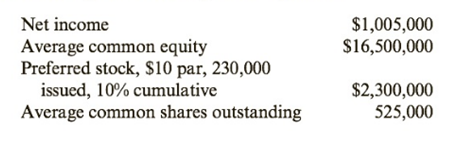Chapter 10, Problem 55BE### Cornerstones of Financial Accounti...

4th Edition
Jay Rich + 1 other
ISBN: 9781337690881

#### Solutions### Cornerstones of Financial Accounti...

4th Edition
Jay Rich + 1 other
ISBN: 9781337690881
Textbook Problem
1 views

# Stockholder Profitability RatiosThe following information pertains to Capital Corporation:Required:Calculate the return on common equity and the earnings per share.

To determine

Concept introduction:

Return on Equity:

Return on Equity is the rate of return earned by the Stockholders on their investment in the company. It is calculated with the help of following formula:

Return on Equity = Net IncomeAverage Stockholders Equity

The Average stock holder’s equity calculated with the help of following formula:

Average stock holders equity=( Beginning stock holders equity + Ending stock holders equity)2

Basic Earnings per share:

The Basic Earnings per share is the amount of net income earned by each common share outstanding. The Earnings per share calculated by with help of following formula:

Basic Earnings per share=Net Income - Preferred DividendWeighted Average Common Shares Outstanding

Net Income available to common stockholder = Net income  Preferred Dividend

To calculate:

The Return on Equity and Earnings per share.

Explanation

The Return on Equity and Earnings per share are calculated as follows:

 Net Income (A) $1,005,000 Average Common Equity (B)$ 16,500,000 Preferred Dividends (C) \$2...

### Still sussing out bartleby?

Check out a sample textbook solution.

See a sample solution

#### The Solution to Your Study Problems

Bartleby provides explanations to thousands of textbook problems written by our experts, many with advanced degrees!

Get Started

#### What is a DBMS, and what are its functions?

Database Systems: Design, Implementation, & Management

#### Explain the difference between over steer and under steer.

Automotive Technology: A Systems Approach (MindTap Course List)

#### Calculate all forces acting on the bar AB described in Prob. 5.1.

International Edition---engineering Mechanics: Statics, 4th Edition